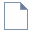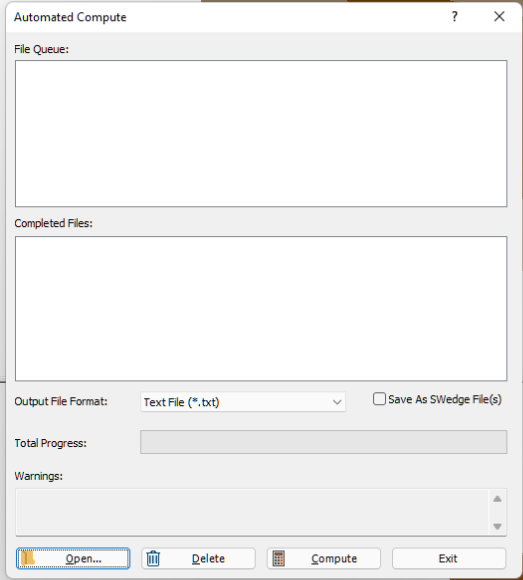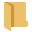# 13 - Automate Probabilistic Analysis

## 1.0 Introduction

The Automate Compute option helps users quickly generate results for several SWedge analysis cases at a time by reading in various model inputs from Excel. In this tutorial, we will familiarize you with the Automate Compute feature by automating the computation of 13 Probabilistic analysis cases, each case with 10,000 samples (i.e., 13 x 10,000 = 130,000 wedges).

Topics Covered in this Tutorial:

• Probabilistic Analysis SWedge Model
• Input Excel File Format
• Pre-processing Input Excel File for Bench Analysis
• Adding Input Excel File(s) to Automate Compute File Queue
• Output SWedge Automate Compute Results File

Finished Product:

The finished products of this tutorial can be found in the Tutorial 13 Automate Compute - Probabilistic Analysis folder, located in the Examples > Tutorials folder in your SWedge installation folder. Inside, you will find:

• Tutorial 13 Automate Compute - Probabilistic_base file.swd7 SWedge model file setup for automation
• Tutorial 13 Automate Compute - Probabilistic_inputs.xlsx Excel input file
• Tutorial 13 Automate Compute - Probabilistic_inputs folder containing the computed results files
• Tutorial 13 Automate Compute - Probabilistic_post-process.xlsx Excel file with all raw combined results data, Excel formula computations, and Excel graph

## 2.0 Creating a New File

1. If you have not already done so, run the SWedge program by double-clicking the SWedge icon in your installation folder or by selecting Programs > Rocscience > SWedge > SWedge in the Windows Start menu.

When the program starts, a default model is automatically created. If you do NOT see a model on your screen:

1. Select: File > NewWhenever a new file is created, the default input data forms valid slope geometry, as shown in the image below.

If the SWedge application window is not already maximized, maximize it now so that the full screen is available for viewing the model.

Before we are ready to run Automate Compute, we must first set up the model environment in the following dialogs:

1. Project Settings
2. Input Data
3. Scale Wedge
This SWedge model file is meant to be used as a base file for automation. It contains the appropriate settings to be used for all automated cases and the engine for computation. It is not meant to be used for model creation and result viewing, as it does not save the model state for every case.

## 3.0 Project Settings

First, set the model Units, Analysis Type, and Block Shape.

1. Select Project Settingsfrom the Analysis menu to open the Project Settings dialog.
2. Set the Units = Metric, stress as MPa
3. Set the Analysis Type = Probabilistic
4. Set the Block Shape = Wedge
6. Set the Number of Samples = 10000
8. Select OK to close the dialog.
The above Project Settings will apply to all cases analyzed in Automate Compute. Each case being analyzed is Probabilistic (i.e. 10,000 sample wedges, as defined) in this case.

## 4.0 Input Data

Next, set the optional input data.

1. Select Input Datafrom the Analysis menu to open the Probabilistic Input Data dialog.
2. Navigate to the Slope page.
Keep all the Statistical Distributions = None and check box controls in the default state. Keep in mind that the following are required input parameters that have to be specified later on in the Input Excel File:
• Slope Dip Mean
• Slope Dip Direction Mean
• Slope Height
• Rock Unit Weight
4. Open the Upper Face tab.
5. Check the Bench Width check box control.
6. Check the Use Slope Dip Direction check box control.
7. Keep all the Statistical Distributions = None.
8. Keep in mind that the following are required input parameters that have to be specified later on in the Input Excel File:

• Upper Face Dip Mean
• Bench Width
9. Open the Joint 1 tab.
10. Set the Orientation Definition Method = Fisher Distribution.
11. Toggle the radio control to Fisher K.
12. Keep Waviness Statistical Distributions = None.
13. Keep in mind that the following are required input parameters that have to be specified later on in the Input Excel File:

• Joint 1 Dip Mean
• Joint 1 Dip Direction
• Joint 1 Fisher K
• Joint 1 Waviness Mean
14. Open the Joint2 tab.
15. Set the Orientation Definition Method = Fisher Distribution.
16. Toggle the radio control to Fisher K.
17. Keep Waviness Statistical Distributions = None.
18. Keep in mind that the following are required input parameters that have to be specified later on in the Input Excel File:

• Joint 2 Dip Mean
• Joint 2 Dip Direction
• Joint 2 Fisher K
• Joint 2 Waviness Mean
19. Open the Strength1 tab.
20. Set the Strength Model = Mohr-Coulomb.
21. Set the Random Variables = Parameters.
22. Set the Cohesion Statistical Distribution = Normal
23. Keep the friction Angle Statistical Distribution = None.
24. Keep in mind that the following are required input parameters that have to be specified later on in the Input Excel File:

• Joint 1 Cohesion Mean, Standard Deviation, Relative Minimum, and Relative Maximum
• Joint 1 Friction Angle Mean
25. Open the Strength2 tab.
26. Set the Strength Model = Mohr-Coulomb.
27. Set the Random Variables = Parameters.
28. Set the Cohesion Statistical Distribution = Normal
29. Keep the friction Angle Statistical Distribution = None.
30. Keep in mind that the following are required input parameters that have to be specified later on in the Input Excel File:

• Joint 2 Cohesion Mean, Standard Deviation, Relative Minimum, and Relative Maximum
• Joint 2 Friction Angle Mean
31. We will not apply Tension Crack, Ponded Water, Joint Water, Seismic Force, or External Forces.
32. Select OK to close the dialog.
You can learn about SWedge input data in detail in Overview of SWedge Input Data.

## 5.0 Input Excel File

Now, let's take a look at the structure of the input Excel file.

1. Obtain the SWedge Probabilistic Excel Template by selecting Analysis > Automate Compute > Probabilistic Excel Template .
2. A Read-Only Excel workbook titled SWedge Automation Template_Probabilistic.xlsx will be automatically opened (if you have Excel installed on your local machine).

The SWedge Probabilistic Input Data Automation Template Excel workbook and worksheets are protected to prevent any accidental modification to the tab structure and headers. The input Excel template contains 13 worksheets for inputting numerical parameters for automation.

3. Create a folder called Tutorial 13 Automate Compute - Probabilistic.
4. Navigate to the SLOPE worksheet.
5. Under the Wedge ID column, provide the names of the wedges. We will name them according to Bench Face Angle (i.e., Slope Dip). Each row in the input Excel file represents one Probabilistic Analysis case in SWedge. We will run a case for each Bench Face Angle ranging from 30 to 90 degrees, by 5-degree increments.
6. Enter the following:
7.  Wedge ID Slope Rock Properties Dip Dip Direction Height Unit Weight Mean Mean BFA 30 30 150 24 0.026 BFA 35 35 150 24 0.026 BFA 40 40 150 24 0.026 ... ... ... ... ... BFA 90 90 150 24 0.026
As set up previously in the SWedge Probabilistic Input Data dialog, only the above input data are required since Slope Length and Statistical Distributions are not applicable. Be aware of units set in SWedge Project Settings when entering data into the input Excel file. The units should be the same for both!

The SLOPE worksheet should look like this:

TIP: Use Formulas or Auto Fill feature in Excel to quickly populate the Wedge IDs.

8. Navigate to the UPPER FACE worksheet.
9. Under the Wedge ID column, provide the names of the wedges.
10. Enter the following:
11.  Wedge ID Upper Face Bench Width Dip Width Mean BFA 30 0 10 BFA 35 0 10 BFA 40 0 10 ... ... ... BFA 90 0 10
As set up previously in the SWedge Deterministic Input Data dialog, the above input data are required since Bench Width and Use Slope Dip Direction are checked. Be aware of units set in SWedge Project Settings when entering data into the input Excel file. The units should be the same for both!

The UPPER FACE worksheet should look like this:

12. Navigate to the JOINT 1 worksheet.
13. Under the Wedge ID column, provide the names of the wedges.
14. Enter the following:
15.  Wedge ID Joint 1 Dip Dip Direction Waviness Fisher Mean Mean Mean Fisher K BFA 30 55 135 0 49 BFA 35 55 135 0 49 BFA 40 55 135 0 49 ... ... ... ... ... BFA 90 55 135 0 49

The JOINT 1 worksheet should look like this:

16. Navigate to the JOINT 2 worksheet.
17. Under the Wedge ID column, provide the names of the wedges.
18. Enter the following:
19.  Wedge ID Joint 2 Dip Dip Direction Waviness Fisher Mean Mean Mean Fisher K BFA 30 75 195 0 49 BFA 35 75 195 0 49 BFA 40 75 195 0 49 ... ... ... ... ... BFA 90 75 195 0 49
20. Navigate to the STRENGTH 1 worksheet.
21. Under the Wedge ID column, provide the names of the wedges.
22. Enter the following:
23.  Wedge ID Joint 1 Cohesion Friction Angle Mean Std. Dev. Rel. Min. Rel. Max. Mean BFA 30 0.015 0.005 0.015 0.015 30 BFA 35 0.015 0.005 0.015 0.015 30 BFA 40 0.015 0.005 0.015 0.015 30 ... ... ... ... ... ... BFA 90 0.015 0.005 0.015 0.015 30

The STRENGTH 1 worksheet should look like this:

24. Navigate to the STRENGTH 2 worksheet.
25. Under the Wedge ID column, provide the names of the wedges.
26. Enter the following:
27.  Wedge ID Joint 2 Cohesion Friction Angle Mean Std. Dev. Rel. Min. Rel. Max. Mean BFA 30 0.015 0.005 0.015 0.015 30 BFA 35 0.015 0.005 0.015 0.015 30 BFA 40 0.015 0.005 0.015 0.015 30 ... ... ... ... ... ... BFA 90 0.015 0.005 0.015 0.015 30

The STRENGTH 2 worksheet should look like this:

We do not need to enter any data in the BASAL JOINT, BASAL STRENGTH, TENSION CRACK, SEISMIC, PONDED WATER, JOINT WATER, or SCALE WEDGE worksheets since those are not applicable.

28. Save the Excel file as Tutorial 13 Automate Compute - Probabilistic_inputs.xlsx to the Tutorial 13 Automate Compute - Probabilistic folder previously created.
Since the SWedge Automation Template_Probabilistic.xlsx file is Read-Only, you must rename and save it in a new location.

We are now ready to automate computations in SWedge by reading from the input Excel file.

## 6.0 Automate from Excel

The Automate Compute dialog allows you to add Excel input automation files to the queue, select an output format, and compute the results.

### 6.1 ADD FILES TO FILE QUEUE

To add multiple files to the File Queue:

1. Select Automate from Excelfrom the Analysis menu to open the Automated Compute dialog.2. Select the Openbutton to open a standard dialog to choose an Excel input file. Navigate to the Tutorial 13 Automate Compute - Probabilistic folder and select the Tutorial 13 Automate Compute - Probabilistic_inputs.xlsx file.

You will see the Excel input file appear under the File Queue list box.

## 7.0 Output Result Files

Results files are automatically generated and saved into the same directory as the input Excel file after Automated Compute is complete. Before we run Compute, first ensure that the Output File Format is set to the desired format. Users can select from either Text File or Excel File format.

### 7.1 TEXT FILE FORMAT

While still in the Automated Compute dialog:

1. Set the Output File Format dropdown to Text File.
2. Select the Compute button to start the automated computing process.
3. The Total Progress can be seen at the bottom of the dialog. When files have finished computing, they will appear in the Completed Files list box.

4. When all files have finished computing, select Exit to close the dialog.

For each input Excel file case, a text file (.txt) containing all results data is generated.

For a Probabilistic Analysis (Tutorial 13 Automate Compute - Probabilistic.xlsx), the results data for each case are output to a separate text file since each case tends to contain hundreds or thousands of wedge samples. Inside each text file, results for all probabilistic wedge samples in the case are outputted following the header row. Each row of data represents one sample wedge. Each data type is separated by a comma (,) for easy parsing.

• Take a look at the ResultsBFA 30.txt result file.
For Slope Dip = 30 deg, no valid wedge geometries are formed.

Note that a total of 13 files with the same format (i.e., ResultsBFA 30.txt, ResultsBFA35.txt, ..., ResultsBFA 90.txt ) have been created; one file per case.

### 7.2 EXCEL FILE FORMAT

Now, we will do the same as before, but this time we will generate the results as an Excel output file format.

1. Select Automate from Excelfrom the Analysis menu to open the Automated Compute dialog.
2. Select the Openbutton to open a standard dialog to choose an Excel input file.
3. Navigate to the Tutorial 13 Automate Compute - Probabilistic folder and select the Tutorial 13 Automate Compute - Probabilistic.xlsx file.
4. You will see the Excel input file appear under the File Queue list box.

5. Set the Output File Format dropdown to Excel File.
6. Select the Save As SWedge File(s) checkbox. This will save each automated compute case as an SWedge file
1. Select the Compute button to start the automated computing process.
2. Since there are already Text File results in the results directory, a popup will appear with the message "Results folder(s) not empty. Duplicated results file(s) will be overwritten. Continue?". Select Yes.
3. When all files have finished computing, select Exit to close the dialog.

For each input Excel file case, an Excel file (.xlsx) containing all results data is generated.

For a Probabilistic Analysis (Tutorial 13 Automate Compute - Probabilistic.xlsx), the results data for each case are output to a separate Excel file since each case tends to contain hundreds or thousands of wedge samples. Inside each Excel file, results for all probabilistic wedge samples in the case are outputted following the header row. Each row of data represents one sample wedge. Each data type is separated by column.

• Take a look at the ResultsBFA 30.xlsx result file.

Note that a total of 13 files with the same format (i.e., ResultsBFA 30.xlsx, ResultsBFA35.xlsx, ..., ResultsBFA 90.xlsx ) have been created; one file per case.

## 8.0 Error Log

As you may have noticed, an Error Log is also generated for each input Excel file. For each input Excel file, an Error Log text file (Automate Compute Error Log.txt) is generated and saved into the same directory as the output file directories. Always check that the Error Log reports "No errors found" before post-processing the raw results data. If any errors do occur as a result of missing (i.e., blank cells) or invalid inputs, the Error Log will indicate which worksheet(s) and cell(s) are problematic.

## 9.0 Bench Analysis Using Post-Processed Results Data

For demonstration purposes, we are going to post-process the results generated from Automate Compute in Excel. We want to generate a plot of [SUM(Volume of Wedges Failed) / SUM(Volume of Valid Wedges)] vs. Bench Angle.

1. Open a new Excel worksheet.
2. Grab the raw data from all Results Excel files.
3. Under the Data tab, select Get Data > From File > From Folder.
4. Browse for the folder containing the Results Excel files.
5. Select Combine > Combine & Load.
6. Select Probabilistic Values and OK to extract the data from each Excel file.
8. Wait while Excel runs the background query. The combined data will contain all the computed data.
9. Post-process the data in Excel.
10. Consider only valid wedges (i.e., Safety Factor is not "N/A")
11. Group wedges by Bench Face Angle (i.e., Slope Dip = 30, 35, ..., 90)
12. Group wedges by Safe (i.e., Safety Factor >= 1) and Failed (i.e., Safety Factor < 1)
13. Sum Weight of Failed Wedges
14. Sum Weight of Valid Wedges
15. Weight Fraction Failed = Sum Weight of Failed Wedges / Sum Weight of Valid Wedges
16. Plot Weight Fraction Failed vs. Bench Face Angle in Excel: